Next: Illustration Up: tr02cb1 Previous: Incorporation of prior knowledge

# Inference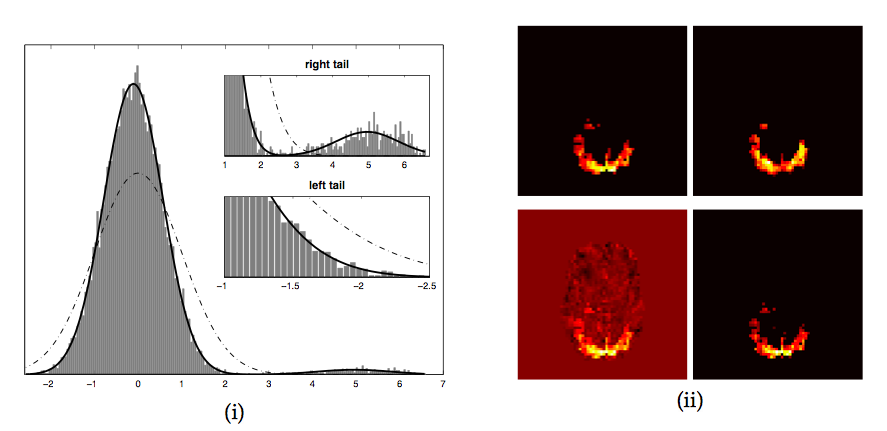After estimating the mixing-matrix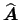, the source estimates are calculated according to equation 6 by projecting each voxel's time course onto the time courses contained in the columns of the unmixing matrix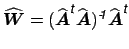.

[McKeown et al., 1998] suggest transforming the spatial maps to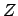-scores (transform the spatial maps to have zero mean and unit variance) and thresholding at some level (e.g,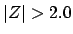). The spatial maps, however, are the result of an ICA decomposition where the estimation optimises for non-Gaussianity of the distribution of spatial intensities. This is explicit in the case of the fixed-point iteration algorithm employed here, but also true for the Infomax or similar algorithms where the optimisation for non-Gaussian sources is implicit in the choice of nonlinearity. As a consequence, the spatial intensity histogram of an individual IC map is not Gaussian and a simple transformation to voxel-wise-scores and subsequent thresholding will necessarily result in an arbitrary and uncontrolled false-positive rate: the estimated mean and variance will not relate to an underlying null-distribution. Figure 1 shows an example where the estimated Gaussian (dash-dotted line) neither represents the 'background noise' Gaussian nor the entire image histogram, and any threshold value based on the expected number of false-positives becomes meaningless with respect to the spatial map.

Instead, consider the estimated residual noise at a single voxel location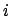: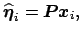where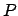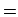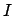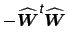is the residual generating projection matrix. In the case where the model order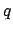was estimated correctly, the columns of the estimated mixing matrixwill span the entire signal space, i.e.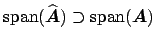so that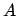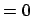. Therefore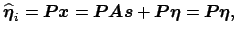i.e. the estimated noise is a linear projection of the true noise and is unconfounded by residual signal. The estimate of the noise variance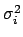at each voxel location is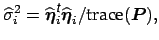which, if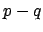is reasonably large, will approximately equal, i.e. equal the true variance of the noise [Jezzard et al., 2001]. We can thus convert the individual spatial IC maps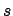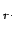into-statistic maps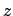by dividing the raw IC estimate by the estimate of the voxel-wise noise standard deviation.

Under the null-hypothesis of no signal and after variance-normalisation, the estimated sources are just random regression coefficients which, after this transformation, will have a clearly defined and spatially stationary voxel-wise false-positive rate at any given threshold level.3While, for reasons outlined above, the null-hypothesis test is generally not appropriate, the voxel-wise normalisation also has important implication under the alternative hypothesis; it normalises what has been estimated as effect (the raw IC maps) relative to what has been estimated as noise and thus makes different voxel locations comparable in terms of their signal-to noise characteristics for a now given basis (the estimated mixing matrix). This is important since the mixing matrix itself is data- driven. As such, the estimated mixing matrix will give a better temporal representation at different voxel locations than at others and this change in 'specificity' is reflected in the relative value of residual noise.

In order to assess the-maps for significantly activated voxels, we follow [Everitt and Bullmore, 1999] and [Hartvig and Jensen, 2000] and employ mixture modelling of the probability density for spatial map of-scores.

Equation 6 implies that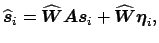i.e. in the signal space defined by the mixing matrix, the additional noise term in equation 2 manifests itself as an additive Gaussian noise term. The same is true after transformation of the intensity values to-scores. We therefore model the distribution of the spatial intensity values of the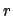th-mapby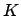mixtures of 1-dimensional Gaussian distributions [Bishop, 1995]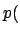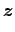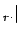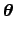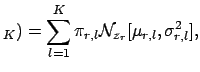(15)

where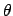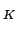denotes the vector of all parameters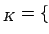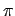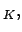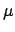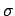} andandare the vectors of themixture coefficients, means and variances. Voxels that are not influenced by a specific time course inwill simply have a random regression coefficient and will be Gaussian distributed. The distribution of intensity values for areas that are influenced by the associated time course, however, can be arbitrary and we will use the fact that the Gaussian mixture model of equation 15 is universal in that any source probability density can be approximated by a sufficient number of mixtures [Bishop, 1995]. As an alternative to this approach, [Hartvig and Jensen, 2000] fits a mixture of one Gaussian and two Gamma distributions to model the probability density of background noise, positive and negative BOLD effects. The model of equation 15 is fitted using the expectation-maximization (EM) algorithm [Dempster et al., 1977]. In order to infer the appropriate number of components in the mixture model we successively fit models with an increasing number of mixtures and use an approximation to the Bayesian model evidence to define a stopping rule (see [Roberts et al., 1998] for details). Our experiments suggest that this typically results in a model with 2-3 mixtures.

In cases where the number of 'active' voxels is small, however, a single Gaussian mixture may actually have the highest model evidence, simply due to the fact that the model evidence is only approximated in the current approach. In this case, however, a transformation to spatial-scores and subsequent thresholding is appropriate, i.e. reverting to null hypothesis testing instead of the otherwise preferable alternative hypothesis testing.

If the mixture model contains more than a single Gaussian, we can calculate the probability of any intensity value being background noise by evaluating the probability density function of the single Gaussian that models the density of background noise. Conversely, we can evaluate the set of additional Gaussians and calculate the probability under the alternative hypothesis of 'activation'4 with respect to the associated time course, i.e. we obtain the estimate of the posterior probability for activation of voxelin the-score mapas [Everitt and Bullmore, 1999]: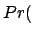activation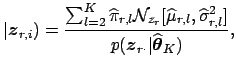where without loss of generality we assume that the first term in the mixture models the background noise. Identification of the Gaussian that models the background is straightforward since it typically coincides with the dominant mode of the intensity histogram.

Figure 3 illustrates the process for a spatial map extracted from a data set with artificial activation introduced into FMRI resting data (see section 5 for details). Voxels with an estimated posterior probability of activation exceeding a certain threshold value are labeled active. The threshold level, though arbitrary, directly relates to the loss function we like to associate with the estimation process, e.g. a threshold level of 0.5 places an equal loss on false positives and false negatives [Hartvig and Jensen, 2000]. Alternatively, because we have explicitly modelled the probabilities under the null and alternative hypothesis, we can choose a threshold level based on the desired false positive rate over the entire brain or at the cluster level simply by evaluating the probabilities under the null and alternative hypotheses.

SubsectionsNext: Illustration Up: tr02cb1 Previous: Incorporation of prior knowledge
Christian F. Beckmann 2003-08-05